1
0
watching
407
views

# What is the conjugate acid ofand the conjugate base ofVideosSolution Video 20 04:15 Expert Answer Was this answer helpful?416 General guidance Concepts and reason The concept used to solve this problem is determined the Conjugated acid-Base. Fundamentals Bronsted-Lowry acid-base system: Acid: According to Bronsted-Lowry; acid is a substance that donates the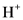(hydrogen ion) or proton. In general Bronsted-Lowry acid is a proton donor. Base: According to Bronsted-Lowry; base is a substance that accepts the(hydrogen ion) or proton. In general Bronsted-Lowry base is a proton acceptor.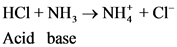Conjugated acid-Base Pairs: The pair of Acid-Base which is differs by a proton is called conjugated Acid-Base pair. The acid of conjugated acid-base pair has one more hydrogen atom from base of conjugated acid-base pair.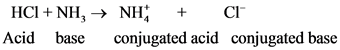After donate one proton from an acid conjugate base will form; thus,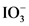is the conjugate base of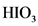.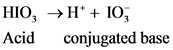After accepts one proton by a base conjugate acid will form; thus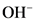is the conjugate acid of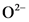.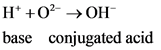Show more  FIRST STEP | ALL STEPS | ANSWER ONLY Step-by-step Step 1 of 4 Part a The conjugate acid of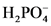will from after accept one proton byas follows: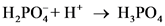After donate one proton from an acid conjugate base will form and after accepts one proton by a base conjugate acid will form. Explanation | Hint for next step The pair of Acid-Base which is differs by a proton is called conjugated Acid-Base pair. The acid of conjugated acid-base pair has one more hydrogen atom from base of conjugated acid-base pair. Step 2 of 4 The conjugate acid ofwill from after accept one proton byas follows:Base Conjugate acid Part a The conjugate acid ofis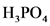. Explanation The pair of Acid-Base which is differs by a proton is called conjugated Acid-Base pair. The acid of conjugated acid-base pair has one more hydrogen atom from base of conjugated acid-base pair. Step 3 of 4 Part b The conjugate base of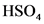will from after donate one proton fromas follows: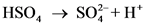Explanation | Hint for next step The pair of Acid-Base which is differs by a proton is called conjugated Acid-Base pair. The acid of conjugated acid-base pair has one more hydrogen atom from base of conjugated acid-base pair. Step 4 of 4 The conjugate base ofwill from after donate one proton fromas follows:Acid Conjugate base Part b The conjugate base ofis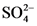. Explanation | Common mistakes The pair of Acid-Base which is differs by a proton is called conjugated Acid-Base pair. The acid of conjugated acid-base pair has one more hydrogen atom from base of conjugated acid-base pair. Answer Part a The conjugate acid ofis. Part b The conjugate base ofis.     Up next for you in Chemistry Part A Although CaCOg isessentially insoluble in purewater, it dissolves slowly inacidic ground water due t…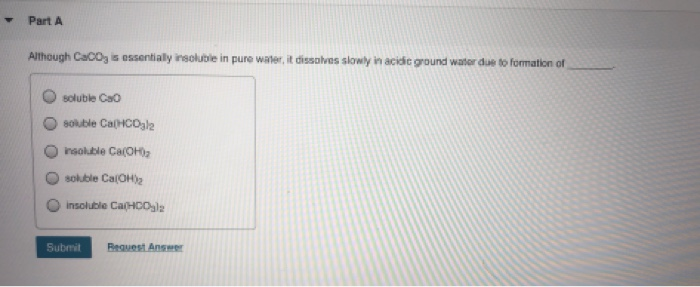See answer Identify the conjugate acidof CO32- and theconjugatebase of HSO4- in thefollowingreaction:HSO4-(aq)+ CO32-(aq)=HCO3-(aq)+SO42-(aq)A. H2CO3 andH2SO4B. HCO3- andSO42-C. HCO3- andH2SO4D. H2CO3 and HSO… See answer See more questions for subjects you study Questions viewed by other students Q: The conjugate acid of HPO42- is H2PO4 H3PO4 PO43- PO42- H2PO4- A: See step-by-step answer 100% (4 ratings) Q: Question 2 1) What is the conjugate base of HCO3−? Express your answer as a chemical formula. 2) What is the conjugate acid of HPO32− ? Express your answer as a chemical formula. 3) Among three bases, X−, Y−, and Z−, the strongest one is Y−, and the weakest one is Z−. Rank their conjugate acids, HX, HY, and HZ, in order of decreasing strength. Rank the acids from strongest to... A: See step-by-step answer 98% (50 ratings)Show more Post a question Answers from our experts for your tough homework questions